### Example: The Foucault Pendulum

A heavy weight on a long cable is suspended from a mount that is free to swing in any direction with very small friction. This is a Foucault pendulum. Its equilibrium position is along the line of a plumb bob, in the direction of effective gravity. With the long cable, the oscillations are small, so the velocity is horizontal and we do everything only to first order in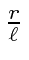.

Its natural to put the origin of coordinates at the equilibrium point, the z axis up, the y axis north, and the x axis east. The motion is in the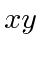plane.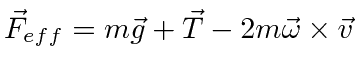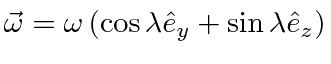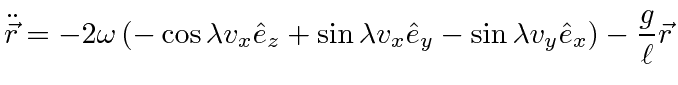We add theequation plus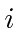times the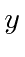equation.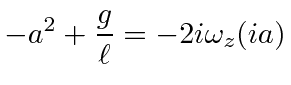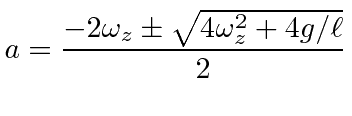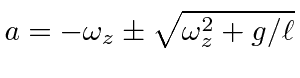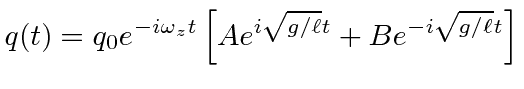Pick the initial conditions on the pendulum to give cosine for the sum of exponentials.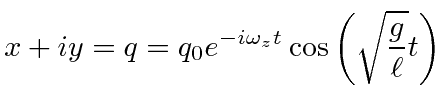The pendulum precesses with an angular frequency.

Jim Branson 2012-10-21